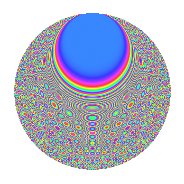# Properties

 Label 162.4.gLevel 162 Weight 4 Character orbit g Rep. character $$\chi_{162}(7,\cdot)$$ Character field $$\Q(\zeta_{27})$$ Dimension 486 Newform subspaces 2 Sturm bound 108 Trace bound 1

# Related objects

## Defining parameters

 Level: $$N$$ $$=$$ $$162 = 2 \cdot 3^{4}$$ Weight: $$k$$ $$=$$ $$4$$ Character orbit: $$[\chi]$$ $$=$$ 162.g (of order $$27$$ and degree $$18$$) Character conductor: $$\operatorname{cond}(\chi)$$ $$=$$ $$81$$ Character field: $$\Q(\zeta_{27})$$ Newform subspaces: $$2$$ Sturm bound: $$108$$ Trace bound: $$1$$ Distinguishing $$T_p$$: $$5$$

## Dimensions

The following table gives the dimensions of various subspaces of $$M_{4}(162, [\chi])$$.

Total New Old
Modular forms 1494 486 1008
Cusp forms 1422 486 936
Eisenstein series 72 0 72

## Trace form

 $$486q + O(q^{10})$$ $$486q - 414q^{18} - 288q^{20} - 216q^{21} + 756q^{23} + 1404q^{26} + 1404q^{27} + 1080q^{29} + 756q^{30} - 864q^{33} - 2484q^{35} - 1152q^{36} - 954q^{38} - 1854q^{41} - 1026q^{45} + 594q^{47} + 2961q^{51} + 2862q^{53} + 2214q^{57} + 1449q^{59} - 1998q^{63} + 7254q^{65} + 9360q^{66} - 5508q^{67} + 1368q^{68} + 4122q^{69} - 1620q^{70} + 1440q^{71} - 576q^{72} - 5472q^{74} - 9000q^{75} + 1620q^{76} - 19584q^{77} - 10656q^{78} + 8424q^{79} - 2880q^{80} - 11520q^{81} - 10656q^{83} - 2448q^{84} + 6480q^{85} - 7200q^{86} - 10944q^{87} + 648q^{88} - 3555q^{89} + 1440q^{90} + 3744q^{92} + 9090q^{93} - 5508q^{94} + 16074q^{95} + 2880q^{96} - 10368q^{97} + 16272q^{98} + 20754q^{99} + O(q^{100})$$

## Decomposition of $$S_{4}^{\mathrm{new}}(162, [\chi])$$ into newform subspaces

Label Dim. $$A$$ Field CM Traces $q$-expansion
$$a_2$$ $$a_3$$ $$a_5$$ $$a_7$$
162.4.g.a $$234$$ $$9.558$$ None $$0$$ $$0$$ $$0$$ $$0$$
162.4.g.b $$252$$ $$9.558$$ None $$0$$ $$0$$ $$0$$ $$0$$

## Decomposition of $$S_{4}^{\mathrm{old}}(162, [\chi])$$ into lower level spaces

$$S_{4}^{\mathrm{old}}(162, [\chi]) \cong$$ $$S_{4}^{\mathrm{new}}(81, [\chi])$$$$^{\oplus 2}$$

## Hecke characteristic polynomials

There are no characteristic polynomials of Hecke operators in the database